# High School Math : Finding Derivatives

## Example Questions

1 2 3 4 5 7 Next →

### Example Question #61 : Finding Derivatives

What is the first derivative of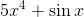?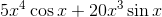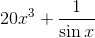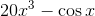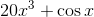Explanation:

Since we're adding terms, we take the derivative of each part separately. For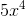, we can use the power rule, which states that we multiply the variable by the current exponent and then lower the exponent by one. For sine, we use our trigonometric derivative rules.

Remember,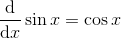.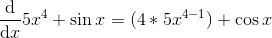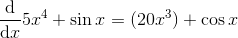### Example Question #62 : Finding Derivatives

What is the second derivative of?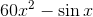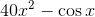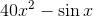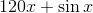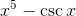Explanation:

To find the second derivative, we need to start by finding the first one.

Since we're adding terms, we take the derivative of each part separately. For, we can use the power rule, which states that we multiply the variable by the current exponent and then lower the exponent by one. For sine, we use our trigonometric derivative rules.

Remember,.Now we repeat the process, but usingas our equation.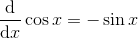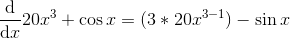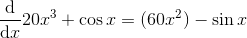### Example Question #3 : Understanding The Derivative Of A Sum, Product, Or Quotient

Which of the following best represents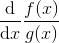?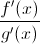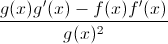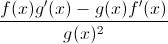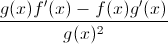Explanation:

The question is just asking for the Quotient Rule formula.

Recall the Quotient Rule is the bottom function times the derivative of the top minus the top function times the derivative of the bottom all divided by the bottom function squared.

Given,the bottom function is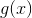and the top function is. This makes the bottom derivative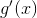and the top derivative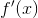.

Substituting these into the Quotient Rule formula resulting in the following.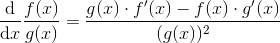1 2 3 4 5 7 Next →